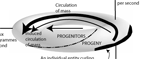﻿ Population Equations | Engenetics: The science of species (by Kofi Busia)

# The population equationsfor biology and ecology

### The Euler equation for biology and for natural selection

μ = dS = (
S
U
)V,{Ni} dU  +  (
S
U
)U,{Ni} dV  +  Σi (
S
Ui
)U,V,{Nj≠i} dU  +  Σi (
S
Ui
)U,V,{Nj≠i} dV

µ is the population's “biological potential” in watts; S is the population's “engeny” in watts per kgs per unit population size, i.e. per “biomole”; Ut = ∫M dt, and is the quantity of biological matter held by the population through its mass flux over that given time interval in kgs; V is “visible presence”, the unit energy density and the inverse of the population's specific Gibbs energy in kgs per joule; N is entity number in the population expressed as a multiple of the unit population size, and so in “biomoles”.

### The Gibbs-Duhem equation for biology and for natural selection

μ = dS = dU  + dH  -  Σiμi ( dvi - dmi )

is the Lagrange multiplier for the population's energy … and also the population's divergence in its mass flux … and also the population's average individual mass flux in kgs per sec.; µ is the population's “biological potential” in watts; S is the population's “engeny” in watts per kgs per unit population size, i.e. per “biomole”;Ut = ∫M dt, and is the quantity of biological matter held by the population through its mass flux over the given time interval in kgs;Ht = ∫P dt, and is the population's total stock of energy held over the given time interval in joules; v is an individual entity's “visible presence” or unit energy density—i.e. that entity's inverse of its specific Gibbs energy, in kgs per joule; m is an individual entity's mass flux, or the mass it maintains at that moment in kgs. per sec.

### Ecology is “the study of the processes systems use to replace their internal energy”.

#### pdt + mdt = dh + du = δq; m > 0 (See explanation of terms and variables)

G  O    T  O    P  A  P  E  RLAW 1: The law of existence
n ≥ 1; δW = (δQ - dU) > 0; m → ∞; > 0

LAW 2: The law of equivalence
[(δW1 = δW2) ∧ (δW2 = δW3)] ⇒ (δW1 = δW3)

LAW 3: The law of diversity
A → 0; FM

LAW 4: The law of reproduction
[(dm̅/dt ≤ 0) ∧ ( > 0)] ⇒ [(dn/dt ≥ 0) ∧ (dA/dt > 0)]MAXIM 1: The maxim of dissipation
[Darwin's theory of competition]
M = nm̅;   ∫dm < 0;   ∇ • M → 0

MAXIM 2: The maxim of number
∇ • H = H/n =

MAXIM 3: The maxim of succession
[Darwin's theory of evolution]
∇ x M = ∂/∂t - ∂n/∂t

MAXIM 4: The maxim of apportionment
∇ x H = ∂/∂t - ∂n/∂t - ∂V/∂t